# Intermediate Organic Chemistry

##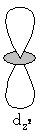I do not like it, and I am sorry I ever had anything to do with it.

Erwin Schrödinger (1887-1961) Austrian physicist. Nobel Prize, 1933. Speaking of quantum mechanics.

Those who are not shocked when they first come across quantum mechanics cannot possibly have understood it.

Niels Henrik David Bohr (1885-1962) Danish physicist.

## That Quantum Stuff

As a student of chemistry it is likely that this chapter will consist of material that you have encountered in your studies for A' level. I am not going to apologise for repeating the information that you find here, a good friend said to me good information is always worth repeating to make sure that it has been fully understood'.

Your comprehension of the way that carbon is unique among elements is paramount in your understanding of the chemistry. For example it is one of the few atoms that undergo catenation.

The structure of an atom of carbon is integral in your insight in how carbon behaves. You should be aware of the structure of the periodic table and how the electrons are arranged for each of the elements. This is the electronic configuration of the element. Each atom has an energy level (shell) known as the principal quantum number (n), n can only have an integral value of 1,2,3 etc.. These energy levels depend on the type of atom. Three further quantum numbers come from the solving of the Schrödinger wave equation i) Angular quantum number (l) values of 0, 1,2 ..n-1 ii) Magnetic quantum number (m) having values of - (l) to + (l) and iii) Spin quantum number (s) having values - ½ and + ½. {see Table 1}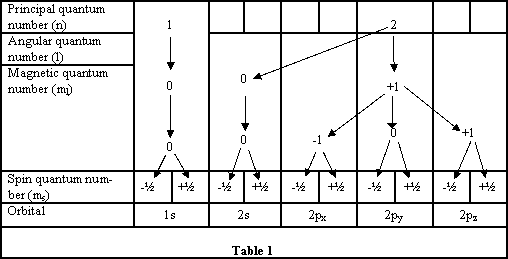Within each energy level there are sub-levels that are called atomic orbitals. This is the region of space where an electron is most likely to be found.

The angular quantum number (i) defines the shape of these orbitals, the atomic orbitals have designations s,p,d,f.. The magnetic quantum number (ii) defines the orientation in space of the orbitals; finally the spin quantum number (iii) gives the value of the spin of the electron with respect to the external field. Quantum numbers (ii) and (iii) are required to identify the electrons in the orbitals, Pauli's exclusion principle states: only two electrons can occupy any atomic orbital, and to do so they must have opposite spins. This is because the electrons have to have different quantum numbers when they occupy these orbitals, and since some levels have more then one orbital in them, the orientation of the electron is required also. The electrons of opposite spins are said to be paired, electrons of like spin tend to get as far from each other as possible. This tendency you will see affects the shapes and properties of molecules.

The atomic orbitals tell us the probability of finding the electron at any particular place within the shape designated; usually this has a 95% probability.

Each atomic orbital has a specific shape: s orbitals are spheres a) and b) in Figure 1 above represent the shapes of all s orbitals regardless of their shell. If we look at the electron density graph of the 1s orbital, (RDF v's distance from the nucleus) Figure 1a, it shows the probability of finding the electron in a Hydrogen atom.

RDF =(wavefunction) 2 x 4pr 2 : RDF=Radial distribution function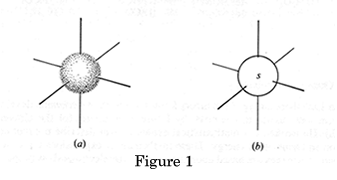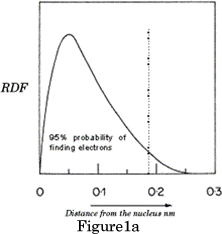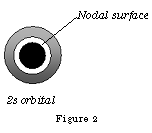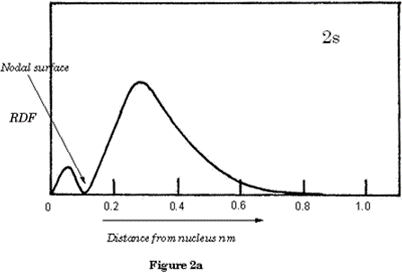Figure 2a shows the electron density of the 2s orbital as a graph; you will observe the nodal surface that appears at  0.1nm. p orbitals are dumb-bell shaped; the shapes represent all p orbitals no matter which shell.

Home